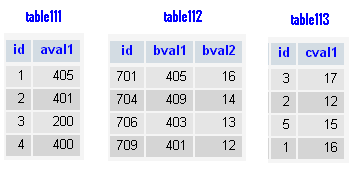# MySQL STRAIGHT_JOIN

## What is STRAIGHT_JOIN in MySQL?

In MySQL an STRAIGHT_JOIN scans and combines matching rows ( if specified any condition) which are stored in associated tables otherwise it behaves like an INNER JOIN or JOIN of without any condition.

"STRAIGHT_JOIN is similar to JOIN, except that the left table is always read before the right table. This can be used for those (few) cases for which the join optimizer puts the tables in the wrong order." - MySQL Manual

MySQL STRAIGHT_JOIN Syntax :

MySQL supports the following JOIN syntaxes for the table_references (A table reference is also known as a join expression.) part of SELECT statements and multiple-table UPDATE and DELETE statements:

```table_references:
escaped_table_reference [, escaped_table_reference] ...

escaped_table_reference:
table_reference
| { OJ table_reference }

table_reference:
table_factor
| join_table

table_factor:
tbl_name [PARTITION (partition_names)]
[[AS] alias] [index_hint_list]
| table_subquery [AS] alias
| ( table_references )

join_table:
table_reference [INNER | CROSS] JOIN table_factor [join_condition]
| table_reference STRAIGHT_JOIN table_factor
| table_reference STRAIGHT_JOIN table_factor ON conditional_expr
| table_reference {LEFT|RIGHT} [OUTER] JOIN table_reference join_condition
| table_reference NATURAL [{LEFT|RIGHT} [OUTER]] JOIN table_factor

join_condition:
ON conditional_expr
| USING (column_list)

index_hint_list:
index_hint [, index_hint] ...

index_hint:
USE {INDEX|KEY}
[FOR {JOIN|ORDER BY|GROUP BY}] ([index_list])
| IGNORE {INDEX|KEY}
[FOR {JOIN|ORDER BY|GROUP BY}] (index_list)
| FORCE {INDEX|KEY}
[FOR {JOIN|ORDER BY|GROUP BY}] (index_list)

index_list:
index_name [, index_name] ...```

Example: MySQL STRAIGHT_JOIN

In the following example no condition have been specified, so, this join has combines each row of left table with all rows in right table.

Code:

``````SELECT table112.id,table112.bval1,table112.bval2,
table111.id,table111.aval1
FROM table112
STRAIGHT_JOIN table111;
```
```

Sample tables:Sample Output:

```+------+-------+-------+------+-------+
| id   | bval1 | bval2 | id   | aval1 |
+------+-------+-------+------+-------+
|  701 |   405 |    16 |    1 |   405 |
|  704 |   409 |    14 |    1 |   405 |
|  706 |   403 |    13 |    1 |   405 |
|  709 |   401 |    12 |    1 |   405 |
|  701 |   405 |    16 |    2 |   401 |
|  704 |   409 |    14 |    2 |   401 |
|  706 |   403 |    13 |    2 |   401 |
|  709 |   401 |    12 |    2 |   401 |
|  701 |   405 |    16 |    3 |   200 |
|  704 |   409 |    14 |    3 |   200 |
|  706 |   403 |    13 |    3 |   200 |
|  709 |   401 |    12 |    3 |   200 |
|  701 |   405 |    16 |    4 |   400 |
|  704 |   409 |    14 |    4 |   400 |
|  706 |   403 |    13 |    4 |   400 |
|  709 |   401 |    12 |    4 |   400 |
+------+-------+-------+------+-------+
16 rows in set (0.00 sec)```

Example : MySQL STRAIGHT_JOIN with condition

In the following example, the STRAIGHT_JOIN performed on a condition and only the matching rows have been retrieves. It behaves like an INNER JOIN or JOIN with condition.

Code:

``````SELECT table113.id,table113.cval1,
table111.id,table111.aval1
FROM table113
STRAIGHT_JOIN table111
ON table113.id=table111.id;
```
```

Sample Output:

```+------+-------+------+-------+
| id   | cval1 | id   | aval1 |
+------+-------+------+-------+
|    1 |    16 |    1 |   405 |
|    2 |    12 |    2 |   401 |
|    3 |    17 |    3 |   200 |
+------+-------+------+-------+
3 rows in set (0.03 sec)```

Key points to remember

Click on the following to get the slides presentation -Previous: RIGHT JOIN
Next: CROSS JOIN

﻿